Courses

# Theory & Procedure, Concave Lens (Focal Length) Class 12 Notes | EduRev

## JEE : Theory & Procedure, Concave Lens (Focal Length) Class 12 Notes | EduRev

The document Theory & Procedure, Concave Lens (Focal Length) Class 12 Notes | EduRev is a part of the JEE Course Physics Class 12.
All you need of JEE at this link: JEE

Our Objective:

To find the focal length of a concave lens using a convex lens by:

• Lens in contact method
• Lens out of contact method

The Theory:

Concave lens

A concave lens is thinner at the center that at the edges. So the light beams passing through the lens are spread out or diverged. Therefore, the concave lens is called a diverging lens. The image formed by a concave lens is virtual and diminished.
Since a concave lens will not produce a real image, a convex lens is used to measure its focal length. There are two methods to find the focal length of a concave lens.

a) Lens in contact method

When a concave lens of focal length f2 is placed coaxially in contact with a convex lens of focal length f1 and if F is the focal length of the combination, then,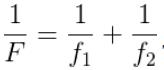Therefore, the focal length of the concave lens,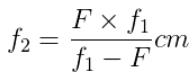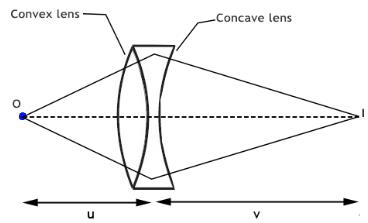b) Lens out of contact method

The real image (I1) formed by the convex lens will act as the virtual object for the concave lens. When concave lens is interposed between the convex lens and the real image I1, the new real image is formed at I2. If u is the distance of the concave lens from the virtual object I1 and v is the distance of the concave lens from the real image I2, then the focal length of the given concave lens is,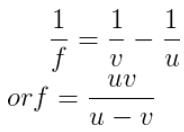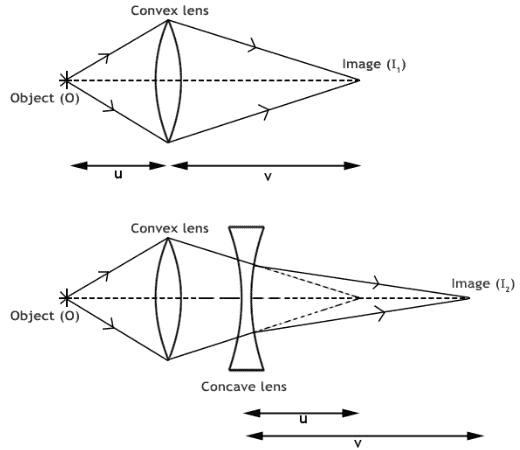Learning outcomes:

• Students list the properties of concave lens.
• Students deduce and apply the lens formula.
• Students identify the two methods used to determine the focal length of concave lens.

Lens in Contact Method

Materials required:

• Illuminated wire gauze
• Lens stand
• Screen
• Metre scale
• A convex lens of short focal length
• Concave lens

Real Lab Procedure:

• Keep the given convex lens of known focal length, f1, in contact with the concave lens whose focal length to be determined. This forms the combination of lenses.
• It is placed between the illuminated wire gauze and the screen at a fixed distance away from the wire gauze. It is taken as u cm.
• The position of the screen is adjusted to get a clear image of the wire gauze on the screen.
• Measure the distance between the combination of lenses and the screen. It is taken as v cm.
• Calculate the focal length of the combination using the formula: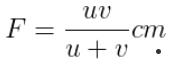• Repeat the experiment by placing the combination of lenses at different distances away from the wire gauze.
• Then calculate the mean value of F.
• Using the values of f1 and F, the focal length of the given concave lens can be calculated using the formula: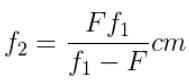Observations:

 SI No. Distance between the combination of lenses and Focal Length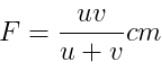Object u (cm) Image v (cm) 1 2 3 4 5

Calculations:

• Focal length of the combination lens, Mean F = …………cm
• Focal length of convex lens, f1 = ……………cm
• Therefore, the focal length of the given concave lens,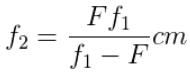Results:

Focal length of the given concave lens by lens in contact method = …………cm

Lens Out of Contact Method

Materials required:

• Illuminated wire gauze
• Two stands
• Screen
• Metre scale
• A convex lens of short focal length
• Concave lens

Real Lab Procedure:

• The given convex lens is mounted on a stand.
• It is placed between the illuminated wire gauze and the screen at a fixed distance away from the wire gauze.
• The position of the screen is adjusted to get a clear image of the wire gauze on the screen at I1.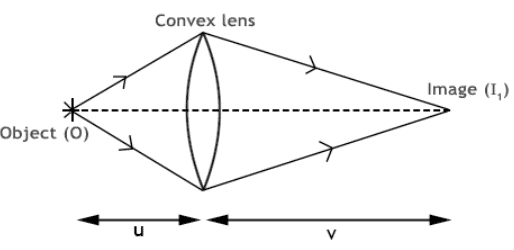• Now, the given concave lens (L) is mounted between the screen and the convex lens without any rearrangement.
• The distance between the screen and the concave lens LI1 is measured as u cm.
• Now, the screen alone is moved back to obtain a clear image Ion the screen.
• The distance between the concave lens and the screen, LI2 is measured as v cm.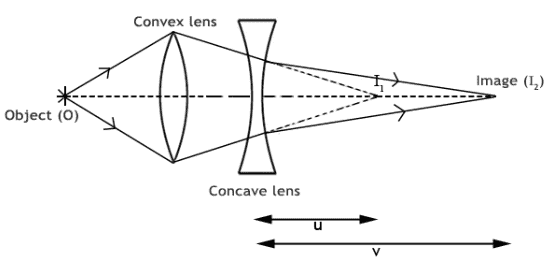• Using the values of u and v, the focal length of the concave lens is calculated using the formula, f= uv/u-v.
• To repeat the experiment, remove the concave lens and bring the screen to the initial position. Then place the concave lens at a different position in between the convex lens and screen and record the values in the tabular column.

Observations:

 SI No. Distance between concave lens and Focal length of concave lensf = uv/u-v cm First image(u cm) Second Image (v cm) 1 2 3 4 5

Calculation:

Focal length of concave lens, Mean f = …………….cm

Result:

The focal length of the concave lens by combination method = …………..cm

Simulator Procedure (as performed through the Online Labs)

Select the method from the drop down list.

Select the concave lens from the drop down list.

Lens in Contact

• Select the distance between the lens (combination of convex lens and concave lens) and the object (wire gauze) using the slider.
• To turn On the wire gauze, click on the ‘Light On’ button.
• Adjust the distance between the lens and the screen using the slider to get a clear image of the wire gauze on the screen.
• Note the value of u and v.
• You can calculate the focal length (F) of combination of lenses using the formula F = uv/(u+v).
• The focal length of convex lens (f1) is shown inside the simulator window.
• You can calculate the focal length of the concave lens using the formula f2 = (f1F/(f1-F).
• You can verify your result by clicking on the ‘Show result’ button.

Lens Out of Contact

• To turn On the wire gauze, click on the ‘Light On’ button.
• Adjust the distance between the convex lens and the screen using the slider to get a clear image of the wire gauze on the screen.
• When a clear image is formed, click on the ‘Insert concave lens’ button to insert the concave lens in between the screen and the convex lens.
• Set the position of the concave lens from the screen using the slider. It is taken as u.
• Adjust the distance between the concave lens and the screen using the slider to get a clear image of the wire gauze on the screen. It is taken as v.
• You can calculate the focal length of the concave lens using the formula f = uv/(u-v).
• You can verify your result by clicking on the ‘Show result’ button.

To redo the experiment, click on the ‘Reset’ button.

Offer running on EduRev: Apply code STAYHOME200 to get INR 200 off on our premium plan EduRev Infinity!

## Physics Class 12

204 videos|288 docs|125 tests

,

,

,

,

,

,

,

,

,

,

,

,

,

,

,

,

,

,

,

,

,

,

,

,

;